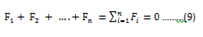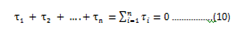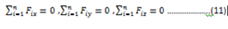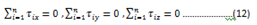A rigid body is said to be in mechanical equilibrium if both its linear momentum and angular momentum are not changing with time, or equivalently, the body has neither linear acceleration nor angular acceleration. This means

• The total force, i.e. the vector sum of the forces, on the rigid body is zero;If the total force on the body is zero, then the total linear momentum of the body does not change with time. Eq. (9) gives the condition for the translational equilibrium of the body.

• The total torque, i.e. the vector sum of the torques on the rigid body is zero,If the total torque on the rigid body is zero, the total angular momentum of the body does not change with time. Eq. (10) gives the condition for the rotational equilibrium of the body.

Eq.(9) and Eq.(10), both, are vector equations. They are equivalent to three scalar equations each. Eq. (9) corresponds toWhere Fix , Fiy , Fiz are respectively the x, y and z components of the forces Fi. Similarly, Eq. (10) is equivalent to three scalar equationsWhere ?ix , ?iy , ?iz are respectively the x, y and z components of the forces ?i.

Eq. (11) and (12) give six independent conditions to be satisfied for the mechanical equilibrium of a rigid body. In a number of problems all the forces acting on the body are coplanar.

Then we need only three conditions to be satisfied for mechanical equilibrium:-

• Two of these conditions correspond to translational equilibrium;

The sum of the components of the forces along any two perpendicular axes in the plane must be zero.

• The third condition corresponds to rotational equilibrium.

The sum of the components of the torques along any axis perpendicular to the plane of the forces must be zero.

A body may be in partial equilibrium, i.e., it may be in translational equilibrium and not in rotational equilibrium, or it may be in rotational equilibrium and not in translational equilibrium.